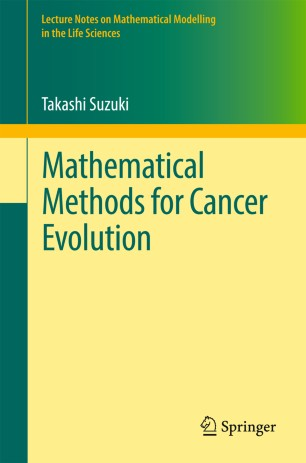# Mathematical Methods for Cancer Evolution

• Takashi SuzukiBook

1. Front Matter
Pages i-vii
2. Takashi Suzuki
Pages 1-11
3. Takashi Suzuki
Pages 13-38
4. Takashi Suzuki
Pages 39-63
5. Takashi Suzuki
Pages 65-133
6. Back Matter
Pages 135-144

### Introduction

The purpose of this monograph is to describe recent developments in mathematical modeling and mathematical analysis of certain problems arising from cell biology. Cancer cells and their growth via several stages are of particular interest. To describe these events, multi-scale models are applied, involving continuously distributed environment variables and several components related to particles. Hybrid simulations are also carried out, using discretization of environment variables and the Monte Carlo method for the principal particle variables. Rigorous mathematical foundations are the bases of these tools.
The monograph is composed of four chapters. The first three chapters are concerned with modeling, while the last one is devoted to mathematical analysis. The first chapter deals with molecular dynamics occurring at the early stage of cancer invasion. A pathway network model based on a biological scenario is constructed, and then its mathematical structures are determined. In the second chapter mathematical modeling is introduced, overviewing several biological insights, using partial differential equations. Transport and gradient are the main factors, and several models are introduced including the Keller‒Segel systems. The third chapter treats the method of averaging to model the movement of particles, based on mean field theories, employing deterministic and stochastic approaches. Then appropriate parameters for stochastic simulations are examined. The segment model is finally proposed as an application. In the fourth chapter, thermodynamic features of these models and how these structures are applied in mathematical analysis are examined, that is, negative chemotaxis, parabolic systems with non-local term accounting for chemical reactions, mass-conservative reaction-diffusion systems, and competitive systems of chemotaxis. The monograph concludes with the method of the weak scaling limit applied to the Smoluchowski‒Poisson equation.

### Keywords

pathway modeling Keller-Segel model mean field theory blowup of the solution reaction-diffusion systems

#### Authors and affiliations

• Takashi Suzuki
• 1
1. 1.Center for Mathematical Modeling and Data ScienceOsaka UniversityToyonakaJapan